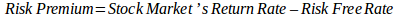# How to Use CARM Model FormulaImage credit: iStock

What is CAPM? Although financial analysts prefer using ratios analysis formula in valuation of assets, the CAPM formula is an essential section in capital budgeting. The Capital Asset Pricing Model (CAPM) describes the link between expected return and systematic risk for assets. It is a vital area in financial analysis; actually, finance became a scientific discipline, fully fledged when William Sharpe invented the CAPM derivation in 1986. Harry Markowitz developed the CAPM model and William Sharpe is among the fine-tuners of the model. Business organizations apply the Capital Asset Pricing Model in calculating costs of capital, generating expected returns for assets given the risk of those assets, and pricing of risky securities. The model explains that the expected return of a portfolio is similar to the risk premium and risk-free security rate. Continue reading for more info provided by our professional profitability index assignment help.

##The CAPM formula ascertains that investors are not compensated for taking unsystematic risks but systematic risks since they can diversify the former. Systematic risks cannot be eliminated because changes in the market impact every asset. Individual assets are sensitive to changes leading to a high risk level. Therefore, CAPM introduced a formula of calculating risk. CAPM formula example:Risk-Free rate: The value of the risk-free rate depends on return rates of Treasury bills since there is nothing like a risk-free stock in financial management. Treasury bills have return rates and values secured by the United States government as they are the yields on government bonds such as U.S. Treasuries. Hence, profit is guaranteed and there is zero risk of losing invested capital.

Market risk premium: It is the return attributed to risk present in the stock market. The market risk premium equals to the risk-free rate subtracted from the stock market’s return rate.Beta: It is a volatility comparison metric. Beta compares the stock’s risk to the larger market’s volatility. A zero beta value is an indication of a perfectly stable security like cash while a beta value of 1 implies that the volatility of the stock market is equal to that of the security. The risk premium multiplied by stock beta gives the expected risk-related return rate which is the risk of the market performance and individual security.

## Differences Between CAPM and APT Models

It is prudent for financial experts, investors, and shareholders to know the stock expected returns before investing. The Arbitrage Pricing Theory and CAPM Pricing Model compares various stocks with regards to the annual yields to allow investors to cautiously choose stocks. The following are the difference between CAPM and APT model:

• CAPM is informative in the short term than in the long term while APT is more accurate in the long term than in the short term.
• The Arbitrage Pricing Theory is mostly used in pricing all risky assets according to their appropriate risks while the Capital Asset Pricing Model finds the required rate of return for all risky assets on the basis of the security’s beta.
• Assumptions of the Capital Asset Pricing Model are restrictive as compared to those of APT. For example, the Arbitrage Pricing Theory permits investors to explain the expected return for a certain asset with a model of their choice while CAPM has a specific model.
• The APT assumes a linear relationship between risk factors while the CAPM assumes the presence of a linear relationship between assets implying that the models cannot predict results where there is no linear relationship.
• CAPM focuses on assets while APT focuses on risk factors. Therefore, the latter is advantageous in that it does not assess risk using a portfolio.

• Systematic risk: CAPM is an effective and reliable method of risk calculation as compared to other models like the Dividend Growth Model since it considers the systematic risk level against the entire stock market which gives companies an opportunity of comparing themselves to the market.
• Diversified portfolio: It eliminates unsystematic risk as numerous investors have diversified portfolios which is a character trait of systematic risks.
• Financial risk and business variability: CAPM is applicable where current businesses differ from financing and business mix during opportunity investigation unlike other required return calculations.
• Ease-of-use: The Capital Asset Pricing Model generates the link between systematic risks and require rate of return which has been used for over 40 years. It is used to develop potential outcomes for provision of confidence in the required rate of return.